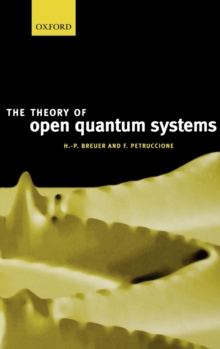# The Theory of Open Quantum Systems Hardback

#### Description

This book treats the central physical concepts and mathematical techniques used to investigate the dynamics of open quantum systems.

To provide a self-contained presentation the text begins with a survey of classical probability theory and with an introduction into the foundations of quantum mechanics with particular emphasis on its statistical interpretation.

The fundamentals of density matrix theory, quantum Markov processes and dynamical semigroups are developed. The most important master equations used in quantum optics and in the theory of quantum Brownian motion are applied to the study of many examples.

Special attention is paid to the theory of environment induced decoherence, its role in the dynamical description of the measurement process and to theexperimental observation of decohering Schrodinger cat states. The book includes the modern formulation of open quantum systems in terms of stochastic processes in Hilbert space.

Stochastic wave function methods and Monte Carlo algorithms are designed and applied to important examples from quantum optics and atomic physics, such as Levy statistics in the laser cooling of atoms, and the damped Jaynes-Cummings model.

The basic features of the non-Markovian quantum behaviour of open systems are examined on the basis of projection operator techniques.

Inaddition, the book expounds the relativistic theory of quantum measurements and discusses several examples from a unified perspective, e.g. non-local measurements and quantum teleportation. Influence functional and super-operator techniques are employed to study the density matrix theory in quantumelectrodynamics and applications to the destruction of quantum coherence are presented. The text addresses graduate students and lecturers in physics and applied mathematics, as well as researchers with interests in fundamental questions in quantum mechanics and its applications.

Many analytical methods and computer simulation techniques are developed and illustrated with the help of numerous specific examples.

Only a basic understanding of quantum mechanics and of elementary concepts of probability theory is assumed.

#### Information

• Format: Hardback
• Pages: 648 pages, numerous figures
• Publisher: Oxford University Press
• Publication Date:
• Category: Probability & statistics
• ISBN: 9780198520634

£127.50

on all orders

###### Pick up orders

from local bookshops